# C For Loop

The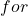loop is a simpler version of the while loop that we learned in the previous lesson. It has the same components as while loop.

## For Loop Basics

The for loop is more popular because all conditions for loop is declared in one place.

Before the for loop starts.

1. Loop variable – It is used for initializing the loop, test condition for loop and increment or decrement the loop.
2. Loop test condition– decide whether to execute the loop body or not.
3. Loop body – contains statements required for performing a task inside the loop.

Logically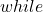loop andloop is same but theloop is lot more simple than theloop. This is because you can initialize, put test conditions and increment or decrement theloop in a single line.

for( initialize counter; test condition; increment or decrement counter)
{
Statements;
}

## Flowchart Symbol of ‘for’ loop

The following symbol is used when we draw a flowchart for a C program that make use of aloop.

## Example Program

In this example program, we are going to a number over and over for 10 times and print the result. This program uses a singleloop.

Your site doesn’t include support for the CodeMirror Blocks block. You can try installing the block, convert it to a Custom HTML block, or remove it entirely.

Install CodeMirror Blocks
Keep as HTML
/* C Program to add a number 10 times */
#include <stdio.h>
int main ()
{
int number, total, i;
number = 2;
total = 0;

for (i=0; i<10; i++)
{
total = total + number;
}

printf ("\n\n\n\n");
printf ("\t\t\tThe total is %d", total);

getch ();
return 0;
}

### Output

The total is 20

## Example Explained

The program has three variables – loop variable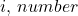and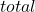and it uses oneloop to repeatedly add the number to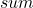until the loop terminates.

First the variables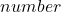and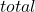are initialized.

total = 0;
number = 2;

The loop variableis used inside theloop to initialize the loop, define the test condition and increment the loop.

for (i =0; i<10; i++)

Since the loop starts at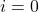, it will run for 10 iteration because the 10th iteration it will terminate. At each iteration, the loop will check the test condition against the current value of.

When the condition for loop is ‘true’, the loop body will execute and add value of variabletorepeatedly.

total = total + number;

The loop adds the10 times and when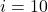, it terminates and prints the value ofas output.

printf ("\t\t\t\t The total is %d", total);

The program ends.

## References